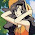## Wednesday, December 30, 2009

### ZOOMING OUT TO MAKE LIFE SIMPLER

This tutorial is just an introduction to make one to think about solving the problem, rather than scratching head for program code directly. My further posts will directly deal with ‘c’ codes.

It is obvious that everyone faces problems in their stages of programming. Each and every puzzle or problems, given are compared by the level of hardness, which actually makes their life miserable.

It is not the programming language that one should go after, but it is the logic that they should strive for.

But then, acquiring the logic from the hidden worlds of brain is not so easy.
So let’s try to make programming life simpler by this short tutorial.

1+3+5+7+9+ ------------------

Now this is not just It is not t a simple “series” which I kept in front of you. Let’s see this as a programmable problem.

This program is no different from our daily life. It is just doing a “work” again and again.

Note:
Every program has a pattern which when revealed, opens the portal of success.
And so, we have to try to find the pattern in this problem.

1+3+5+7+9+--------

Other than 1, every element obtained here has a reason i.e., “2” is added to get the new term or element.

Hence, we get the pattern now

1st term: 1
2nd term: 2
3rd term: 3

Let ‘n’ be the number of the term in the series.

So, n=1 --> 1
n=2 --> 3
n=3 --> 5

Now we need to search for the logic which solves the above scenario.
And it would be (2n-1)

As I said earlier, we have not just solved a series but actually saw how a how a simple problem can be represented through a pattern.

And now, we will pass on to the main section of viewing the problem from the view of program.

1+1+3+4+5+7+7+-----------------

After solving the first problem, it is natural to search for a particular pattern for all the terms in this problem also.

But it is a bit different case in finding the pattern here.
Observe that the given problem comprises of two series.

1+3+5+7+-------
1+4+7+10+--------

A program or problem may deal with many sub problems. So we need to divide them initially. And final solution would appear on joining these sections.

Hence, (2n-1) + (3n-2) is final solution.
And so we saw that a hidden pattern(chunks of modules !) solves the whole logic and a problem with many sub problems have to be divided first.

#### 1 comment:

1.